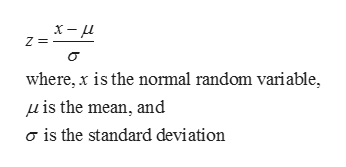# Given a standardized normal distrubtion (with a mean of 0 and a standard deviation of 1, as in Table E.2) what is the probability thata. Z is less than 1.57?b. Z  is greater than 1.84?c. Z is between 1.57 and 1.84?d. Z is less than 1.57 or greater than 1.84?

Question
394 views

Given a standardized normal distrubtion (with a mean of 0 and a standard deviation of 1, as in Table E.2) what is the probability that

a. Z is less than 1.57?

b. Z  is greater than 1.84?

c. Z is between 1.57 and 1.84?

d. Z is less than 1.57 or greater than 1.84?

check_circle

Step 1

Standard normal distribution:

The standard normal distribution is a special case of normal distribution, in which the mean of the distribution is 0 and standard deviation of the distribution is 1. The Z-scores can be calculated using the given formula.help_outlineImage Transcriptioncloseх— и where, x is the normal random variable is the mean, and g is the standard deviation fullscreen
Step 2

a. Probability of Z is less than 1.57:

The probability of Z is less than 1.57 is found to be 0.9418 from the calculation given below.

Step 3

b. Probability of Z is greater than 1.84:

The probability of Z is greater than 1.84 is fou...

### Want to see the full answer?

See Solution

#### Want to see this answer and more?

Solutions are written by subject experts who are available 24/7. Questions are typically answered within 1 hour.*

See Solution
*Response times may vary by subject and question.
Tagged in

### Other